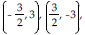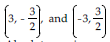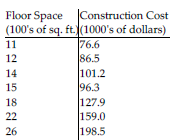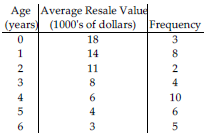/
/
/
241) f(x, y) = x2 + xy + y2 the
Not my Question
Flag Content

# Question : 241) f(x, y) = x2 + xy + y2 the : 1757205

241) f(x, y) = x2 + xy + y2 on the square -3 x, y 3

A) Absolute maximum: 9 at (3, -3) and (-3, 3); absolute minimum: 0 at (0, 0)

B) Absolute maximum: 9 at (3, -3) and (-3, 3); absolute minimum: 27/4 atC) Absolute maximum: 27 at (3, 3) and (-3, -3); absolute minimum: 9 at (3, -3) and (-3, 3)

D) Absolute maximum: 27 at (3, 3) and (-3, -3); absolute minimum: 0 at (0, 0)

242) f(x, y) = 8x + 4y on the trapezoidal region with vertices (0, 0), (1, 0), (0, 2), and (1, 1)

A) Absolute maximum: 12 at (1, 1); absolute minimum: 8 at (1, 0)

B) Absolute maximum: 8 at (0, 2); absolute minimum: 0 at (0, 0)

C) Absolute maximum: 12 at (1, 1); absolute minimum: 0 at (0, 0)

D) Absolute maximum: 16 at (2, 0); absolute minimum: 8 at (1, 0)

243) f(x, y) = 6xy2 + 9xy on the trapezoidal region with vertices (0, 0), (1, 0), (0, 2), and (1, 1)

A) Absolute maximum: 15 at (1, 1); absolute minimum: 0 at (0, 0)

B) Absolute maximum: 15 at (1, 1); absolute minimum: 6 at (1, 0)

C) Absolute maximum: 18 at (0, 2); absolute minimum: 0 at (0, 0)

D) Absolute maximum: 12 at (2, 0); absolute minimum: 6 at (1, 0)

244) f(x, y) = x2 + 16x + y2 + 18y + 3 on the rectangular region -1 x 1, -2 y 2

A) Absolute maximum: 79 at (2, 2); absolute minimum: 3 at (0, 0)

B) Absolute maximum: 79 at (2, 2); absolute minimum: -44 at (-1, -2)

C) Absolute maximum: 60 at (1, 2); absolute minimum: -44 at (-1, -2)

D) Absolute maximum: 60 at (1, 2); absolute minimum: 3 at (0, 0)

245) f(x, y) = x2 + y2 on the diamond-shaped region |x| + |y| 8

A) Absolute maximum: 64 at (8, 0) and (0, 8); absolute minimum: 0 at (-8, 0) and (0, -8)

B) Absolute maximum: 64 at (8, 0), (-8, 0), (0, 8), and (0, -8); absolute minimum: 32 at 4, 4 , 4, - 4 , - 4, 4 , and - 4, - 4 ,

C) Absolute maximum: 64 at (8, 0), (-8, 0), (0, 8), and (0, -8); absolute minimum: 0 at (0, 0)

D) Absolute maximum: 32 at 4, 4 , 4, - 4 , - 4, 4 , and - 4, - 4 ; absolute minimum: 0 at (0, 0)

246) Find the least squares line through the points (1, -6) and (2, -9).

A) y = -3x - 21 B) y = -15x - 21 C) y = -3x - 3 D) y = -15x - 3

247) Find the least squares line through the points (1, -42), (2, -24), and (3, -12).

A) y = 15x - 56 B) y = 15x - 72 C) y = -27x - 72 D) y = -27x - 56

248) The table below summarizes the construction cost of a set of homes (excluding the lot cost) along with the square footage of the home's floor space. Find a linear equation that relates the construction cost in thousands of dollars to the floor space in hundreds of square feet by finding the least squares line for the data.A) y = 7.87x - 13.08 B) y = 7.97x - 13.54 C) y = 8.13x D) y = 8.13x - 12.79

249) Amarillo Motors manufactures an economy car called the Citrus, which is notorious for its inability to hold a respectable resale value. The average resale value of a set of 1998 Amarillo Citrus's is summarized in the table below along with the age of the car at the time of resale and the number of cars included in the average. Fit a line of the form ln(V) = m?a + b to the data, where V is the resale value in thousands of dollars and a is the age of the car in years..A) ln(V) = 0.314a - 2.986 B) ln(V) = 0.299a - 2.951 C) ln(V) = 0.315a D) ln(V) = -0.303a + 2.953

250) f(x, y) = 8x2 + 5y2, x2 + y2 = 1

A) Minimum: 5 at (0, ±1); maximum: 0 at (0, 0)

B) Minimum: 5 at (±1, 0); maximum: 8 at (0, ±1)

C) Minimum: 5 at (±1, 0); maximum: 0 at (0, 0)

D) Minimum: 5 at (0, ±1); maximum: 8 at (±1, 0)

## Solution 5 (1 Ratings )

Solved
Mathematics 3 Years Ago 56 Views